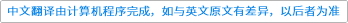Journal of Computational Science ( IF 2.644 ) Pub Date : 2021-04-07 , DOI: 10.1016/j.jocs.2021.101363
Davide Dapelo, Stephan Simonis, Mathias J. Krause, John Bridgeman

Traditional Lattice-Boltzmann modelling of advection–diffusion flow is affected by numerical instability if the advective term becomes dominant over the diffusive (i.e., high-Péclet flow). To overcome the problem, two 3D one-way coupled models are proposed. In a traditional model, a Lattice-Boltzmann Navier–Stokes solver is coupled to a Lattice-Boltzmann advection–diffusion model. In a novel model, the Lattice-Boltzmann Navier–Stokes solver is coupled to an explicit finite-difference algorithm for advection–diffusion. The finite-difference algorithm also includes a novel approach to mitigate the numerical diffusivity connected with the upwind differentiation scheme.

The models are validated using two non-trivial benchmarks, which includes discontinuous initial conditions and the case ${\mathrm{Pe}}_{\mathrm{g}}\to \infty$ for the first time, where ${\mathrm{Pe}}_{\mathrm{g}}$ is the grid Péclet number. The evaluation of ${\mathrm{Pe}}_{\mathrm{g}}$ alongside $\mathrm{Pe}$ is discussed. Accuracy, stability and the order of convergence are assessed for a wide range of Péclet numbers. Recommendations are then given as to which model to select depending on the value ${\mathrm{Pe}}_{\mathrm{g}}$—in particular, it is shown that the coupled finite-difference/Lattice-Boltzmann provide stable solutions in the case $\mathrm{Pe}\to \infty$, ${\mathrm{Pe}}_{\mathrm{g}}\to \infty$.Lattice-Boltzmann耦合模型可在多种佩克利数上进行平流-扩散流

down
wechat
bug